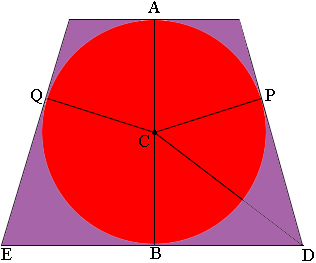SEARCH HOMEMath Central Quandaries & QueriesQuestion from priyam, a student: A circle is inscribed inside an isosceles trapezoid (with parallel sides of length 18 cm and 32 cm) touching all its four sides. find the diameter of the circle. thanks for help!!Priyam,

I can give you some hints.

Here is my diagramThe sides of the trapezoid are tangent to the circle at $A, B, P \mbox{ and } Q.$ An important fact is that at each of these points the radius and tangent are perpendicular.

$ACB$ is a straight line. Do you see why? Since the trapezoid is isosceles the symmetry of the diagram tells us that $B$ is the midpoint os the side $DE$ and hence you know the length of $BD.$

Triangles $PCD$ and $CBD$ are congruent. Do you see why? Thus you know the length of $PD.$

Can you complete the problem now?

Write back if you need more help.

PennyMath Central is supported by the University of Regina and The Pacific Institute for the Mathematical Sciences.